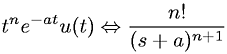Equations > Signal Processing > Laplace Transform Pairs > Laplace transform involving the unit step function and an exponential

### Laplace transform involving the unit step function and an exponentialLatex Code:

MathML Code:

 ${t}^{n}{e}^{-\mathrm{at}}u\left(t\right)⇔\fracn!\left(s+a{\right)}^{n+1}$

MathType 5.0: